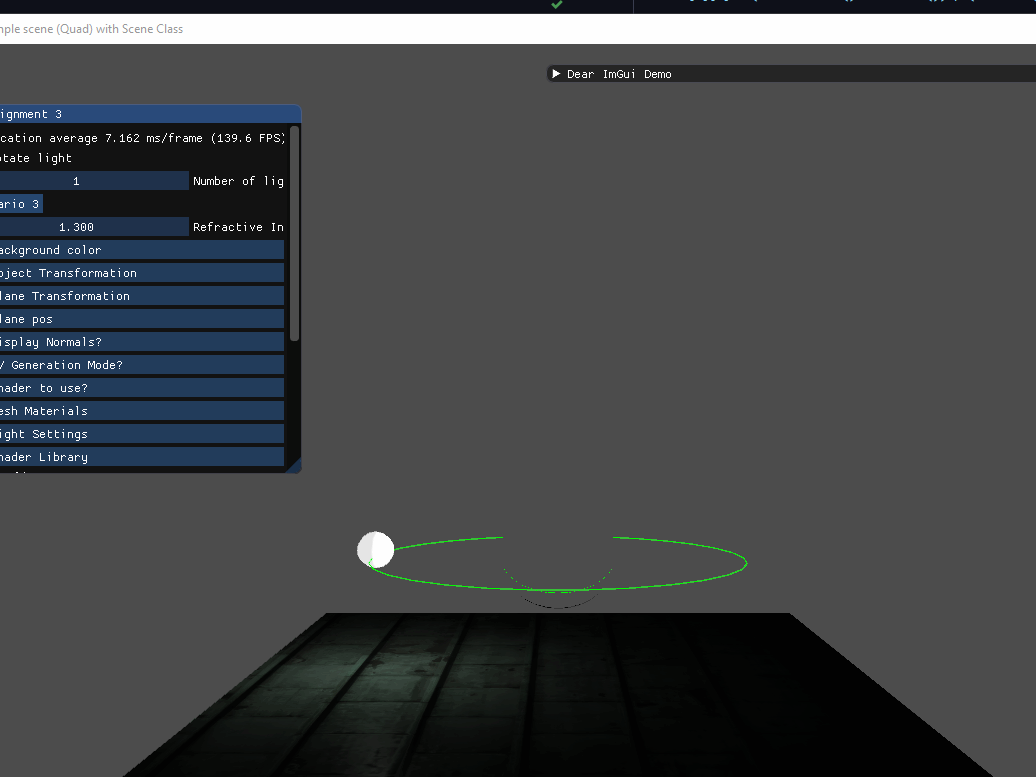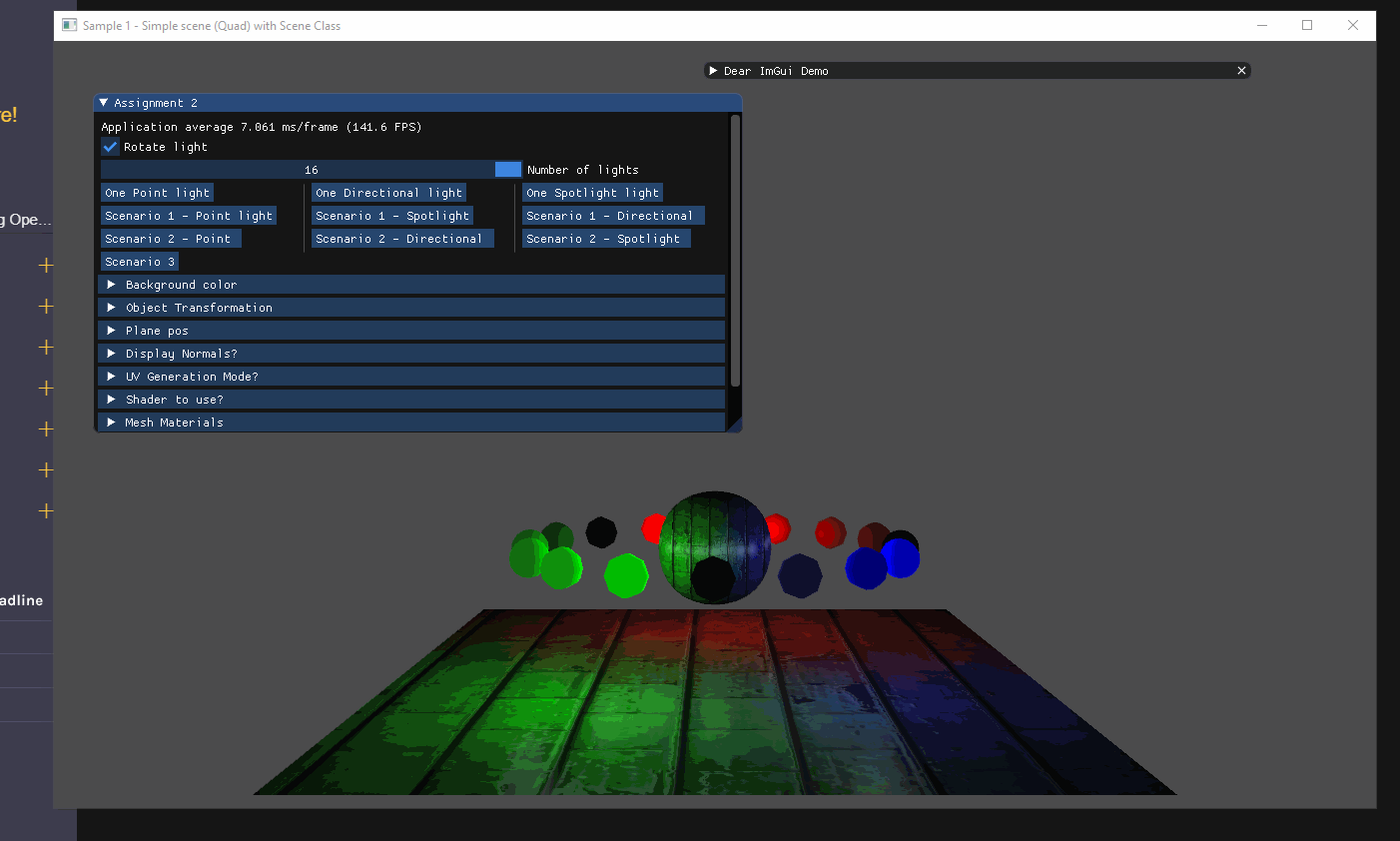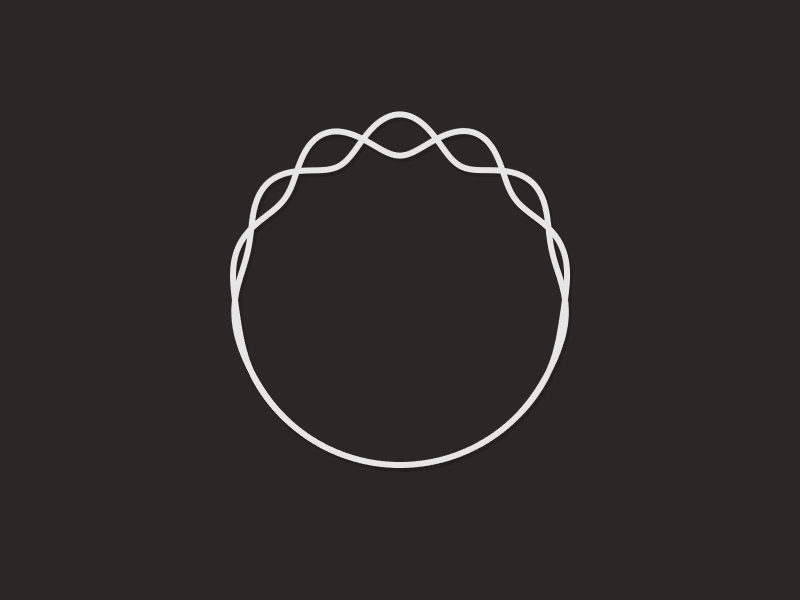top of page

# Perspective Engine

Project Type:

Technology:

Platform(s):

Status:

Development Period:

C++, OpenGL, 3D Animations, Physics

Windows

Complete

08/2020 - 12/2021

Perspective is a game engine I wrote. It started out as just an OpenGL renderer but has evolved to fit the needs of my assignments. The project is built using CMake and uses ImGUI as an interface.## Rigid body Physics Simulation

I wrote a box physics simulation based on Erin Catto's Box2D. It is an impulse-based physics engine, meaning it fulfills the constraints using tiny impulses.

There are three main difficulties in writing this physics engine.

1. Integration of forces to velocity to position

2. Detecting when a collision happens

3. Having the correct response to the collision

It turns out for modern run-time physics; a constraint system works best for collision response. Hence I coded three constraints; friction constraint, penetration constraint, and a joint constraint. Next, I applied tiny impulses to my object's velocity until the conditions were fulfilled.

For collision, I used Separating Axis Theorem (SAT) for collision detection while using Gilbert–Johnson–Keerthi distance algorithm (GJK) for penetration depth and penetration point. From there, I built a list of contacts and used the best contact point. Finally, this contact point is sent to the constraint system to determine how to use it.

To code each constraint, I relied on Erin Catto's GDC talks. The basic premise is to find the impulse to adjust the velocity and keep applying until a decent solution is found. It is important to apply a bias and a slur impulse as well, to prevent sinking.

I imported Assimp to load 3D models. While Assimp handled the loading, I still had to generate the Vertex Buffer Array (VBO) and Vertex Array Object (VAO) for OpenGL to render.

Below is a sample I have. I am loading a factory split into several different models, each averaging around 300K triangles.

I initially created the engine to be a renderer to experiment with different rendering techniques. Thus it was imperative to have a fast iteration process modifying shader codes.

The preprocessor I wrote causes a problem when a GLSL error is displayed; the error does not correspond to the correct line since I manually preprocess the code. I then coded an ImGUI shader to indicate the error and the resulting file.

For example, take we have the below shader code.

And then in engine...When I fix this error in my editor (not shown due to recording limitations), the engine auto-reloads it and applies the fix.

## Deferred Rendering - Lighting

I used modern rendering techniques to render lights in a deferred manner. I used the Blinn-Phong lighting model and captured the necessary data to my GBuffer, and in my final run calculate the light for each pixel and render it.

Deferred Rendering is the technique of using multiple render passes to construct a final output pass. This is especially useful for lighting for example, where it will be too computationally heavy to compute each object's brightness if there were 1000 lights in the scene. It would make much more sense to compose the scene first, and then apply the 1000 lights at one go.

In my implementation, I have a geometry pass where I gather the data required for the lighting pass. This data is gathered into a buffer known as the GBuffer. Below I have a visualization of the GBuffer and its data.

After the geometry pass, I then apply my light pass where it lights up each pixel. Finally, I added an environment pass to add in the skybox.GBuffer storage displayFinal result using Blinn-Phong lighting (models were not texured)## Data Structures For Collision

Part of the physics work was to code a Broadphase detection for collision. This is just a means to speed up the entire physics system. The premise is to use a cheap and inaccurate detection to determine if we want to do expensive but accurate collision detection for objects that may collide.

For this project, I coded two different types of data structures for this. I first coded a binary tree, and then an oct tree. They are both displayed below.

## Binary Tree

A binary tree attempts to cut a scene in half as much as possible. It keeps doing this until x amount of the scene is in the leaf node.

The crux of this algorithm is how to determine the line that cuts the scene in half. For my purposes, I used a "best score from 10 random splits" as I found that to be the most performant (keeping 60 FPS while giving decent results).

The scoring method I used is based on how many vertices are in front, behind, or straddling the line.

float score = K * numStraddling + (1.0f - K) * std::abs(numInFront - numBehind);

Where K is a blend factor I specify for optimizing for splits.

I applied this algorithm to my models and the results are below. Each color represents a different leaf node.## Oct Tree

An oct tree is similar to a binary tree. First, we build a massive box that contains our entire scene. If the box contains more objects than it can hold, it splits itself into eight equal-sized boxes. Keep recursively doing this until none of the boxes holds more objects than its capacity.## 3D Animation - Interpolating Bones

As mentioned before, I added Assimp support to my project. Using Assimp, I loaded the skeletal frame data for the FBX animation.

The idea behind the skeletal animation is not particularly hard, but executing it proved a challenge. The FBX files give a timeline of the Translate, Rotate, and Scale of the bone in its bone space. Thus it is necessary to find a matrix that converts the bone's space to model space.

For the interpolation of the Translate, Rotate, and Scale, I experimented with several techniques.

• Translate

• Linear Interpolation (Lerp)​

• Incremental Linear Interpolation​​ (iLerp)

• Exponential Interpolation

• Rotate​

• Spherical Interpolation (Slerp)​

• Incremental Slerp (iSlerp)

• Scale​

• Lerp

• iLerp

Another technique I used was to use a Vector-Quaternion-Scale (VQS) format to store the Translate, Rotate, and Scale information. Usually, such information is stored as a homogenous matrix, but storing as a VQS lowers the size of the data structure from 16 floats to 8 floats. ​​

VQS also simplifies the mathematics as a product of two quaternions also gives its movement, but you get to avoid all the sin() cos() mess of a homogenous matrix. This should improve computational speeds at runtime.

A further improvement I made was to apply the incremental techniques to the VQS system. By identifying how many steps I need to make, I can find the difference between each VQS step. This optimizes the algorithm even more as only the difference requires sin() and cos() to be found. After initialization, the iVQS just adds on itself and is pretty cheap computationally.

## Code Snippet

Below is the code for the VQS data structure, as well as the implementation for iVQS.

## Path Generation and Traversal

As part of my animation work, I created a Path Generator. My path generator takes in certain points that the developer wants to hit, and generates a Bezier curve that hits all four points.

Mathematically, we are taking every two points in the set and generating a bezier curve from four points by adding in the first and the last point. After that, we concatenate them all together to form one single line.

A wrinkle in using this line is the fact that the bezier curve is a Bernstein polynomial. Game developers however only really care for a [0,1] type of function. Usage would probably be like

vec3 point = path.getPoint(0.5); // Get the point in the middle of the path

Hence an internal table that maps arclength of the curve to a point on the path is used. I implemented the table as a binary tree. When getPoint() is requested, I use the tree to find the closest point, and then lerp till it's fully 50% of the arclength through.

The video below shows my model going through a path generated from the GUI. The model eases in to a constant velocity, and then eases out back to 0